Education ∪ Math ∪ Technology

# Tag: math(page 2 of 10)

I am presenting in Hope, British Columbia today, on the topic of Math in the Real World. Here are my presentation slides.

You will probably notice that sections 6 and 7 of my presentation are not completely focused on the topic of “math in the real world” but I feel like they are such important concepts for mathematics teachers to understand that I needed to include them in my presentation.

I covered a couple of my colleague’s classes yesterday so he could attend a math conference. The afternoon class was a somewhat boisterous grade 10 group. I was asked to teach students how to find the greatest common factor, and if I had time, introduce them to more general factoring techniques.

I decided that the greatest common factor is a topic students find relatively easy, and so I just showed some examples of how to do it (actually, I drew the "how to" out of the class by asking them questions, but this is my standard technique) after verifying that they understood the distributive principle. I then assigned some practice problems, which then each student wrote their solution up on the board, and we discussed. I then showed students a couple of different techniques for multiplying binomials (like (x+2)(x+3) for example).

Next, I put up the following 4 questions.

1. x2 + 7x + 12

2. 2x2 + 7x + 3

3. x2 – 25

4. x3 + 8

I asked students to try and figure out how to write these expressions as one set of brackets times another, just like with the example from before, but I suggested to them that what we are trying to do is undo the distributive rule.

I went around the room and encouraged students, gave them hints when they needed them, asked them questions to prod their thinking, and observed their problem solving strategies. Students were engaged in the problem solving activity for a good 30 minutes. Once some of the students’ attentions started to wane a bit, I gave them a sheet with a description of how to do factoring by grouping and some problems to work on the back.

A group of students though really dove into question 4, which, as you may notice is actually quite a bit more difficult than the other three problems. I ended up having to give students two hints: I told them that the expression broke into two factors, one of which was (x+2) and the other of which was three terms long. The group of students worked feverishly on solving the 4th problem for a good twenty minutes, and then all of a sudden, one of the girls in the group leapt out of her seat and screamed, "I GOT IT!! YES!!" I circled around to see if she had the right answer, asked her how she was so sure it was right (she had multiplied everything back through using the distributive rule), and then gave her group x3+27 to solve (which she did quickly) and then x3 + a3 to solve.

At 5:30pm that night, I received an email from the girl, excitedly telling me how she had an inspiration while she was on the bus home on how to solve the general question, and had then figured out the general formula for how to factor a sum of cubes.

I emailed her back and congratulated her on becoming a mathematician.

I played ultimate tonight, and we usually keep score with shoes. Our normal scoring system is to count in base 5. Tonight, I tried to use binary, but at half-time I switched back to base 5 when most of our team struggled to read our score quickly.

I took some pictures of the arrangement of shoes during the game (when I wasn’t playing).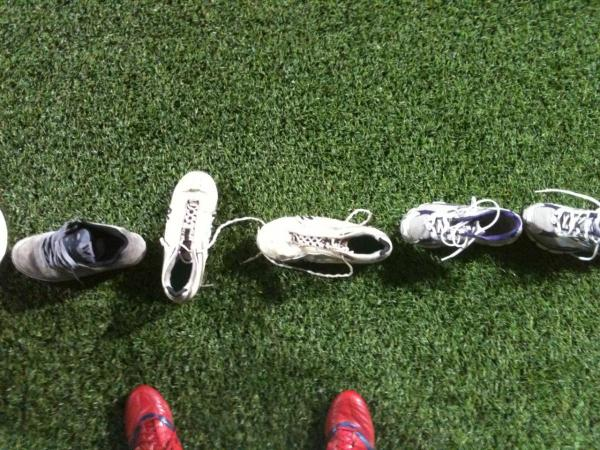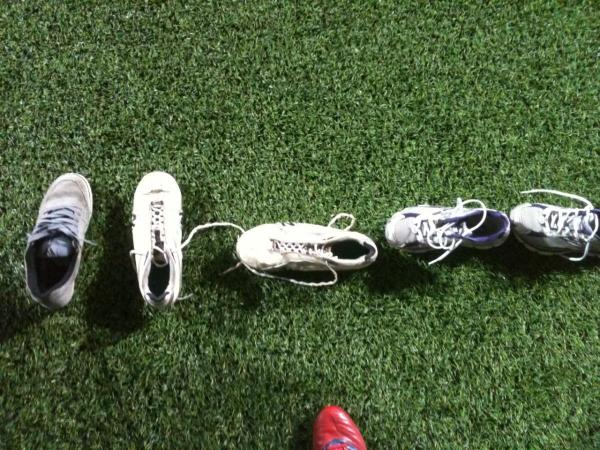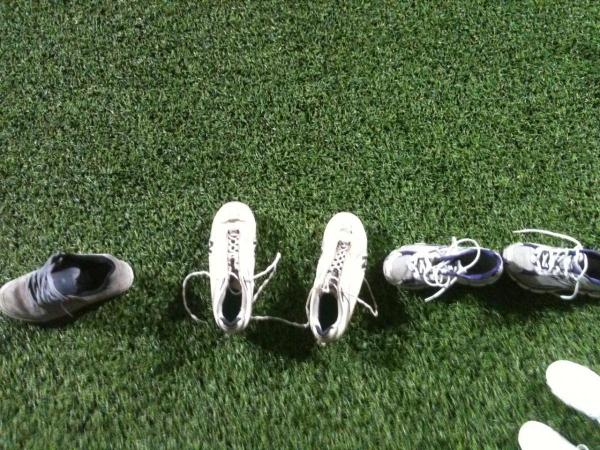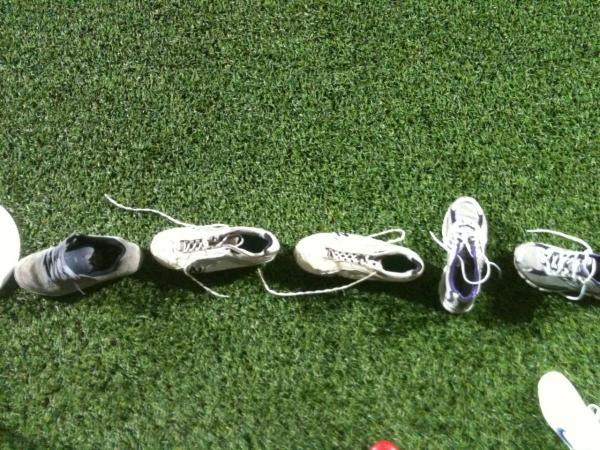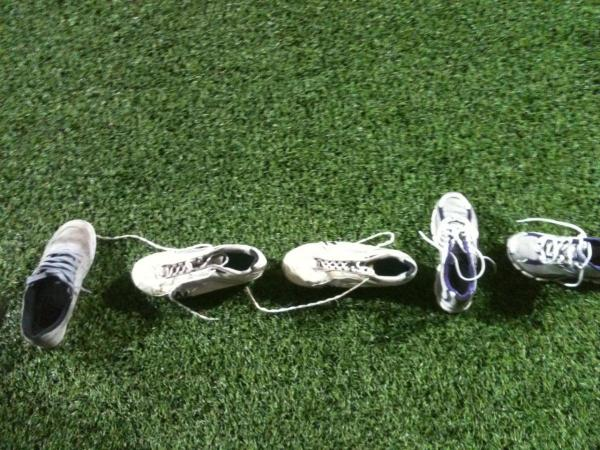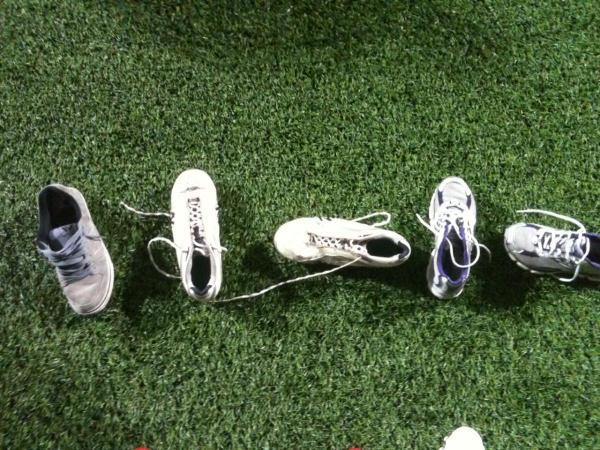I can imagine some investigations could be made out of these photos.

• Given the numbers associated with each photo, try and determine how to count in this number system,
• More challenging: From these photos, try and determine the missing numbers.

If you want a project that might take a while:

• Design a scoring system using shoes. It should be easy to maintain and not require too many shoes.

Our department had a meeting recently where we discussed the need for more investigative approaches in our teaching. We all use investigative approaches at least part of the time, but some of us disagreed about whether it was possible to approach every topic with an investigation.

One of the specific topics that came up was "the rules for multiplying positive and negative integers". Here are some ideas we came up with:

1. Give students calculators and have them try out different calculations with different signs. They are likely to quickly discover the "rules" for multiplying and dividing positive and negative numbers, but I am not sure if they will understand why the rules work. Still, it’s a step in the right direction toward student discovery.

2. Have students generate patterns when they are multiplying positive and negative numbers like so:
3 x 4 = 12
3 x 3 = 9
3 x 2 = 6
3 x 1 = 3
3 x 0 = 0

Prompt the students to see what patterns they notice about this list of multiplications. Ask them to extend the patterns another couple of rows. They will have hopefully noticed that the second number in the multiplication is decreasing by one, and that the answer is decreasing by three each time. They will need to have a good understanding of multiplication, subtraction, and negative numbers to be able to be successful in this investigation. This will be an excellent opportunity to formatively assess students on their understanding of negative numbers to see if they can extend this pattern to 3 x -1 = -3.

Have students repeat this process for another set of similar multiplications but perhaps with the pattern flipped around slightly. For example:

2 x 5 = 10
1 x 5 = 5
0 x 5 = 0

You can then set up other similar patterns which should result in the students developing similar rules for other forms of multiplication.

5 x -3 = -15
4 x -3 = -12
3 x -3 = -9
2 x -3 = -6
1 x -3 = -3
0 x -3 = 0

There are some advantages of this approach. First, the students will see that the rules for multiplying positive and negative integers come somewhere; they are in essence necessary to preserve the internal inconsistency of multiplication with these patterns. Second, the students will necessarily get some practice multiplying some of the numbers together with a purpose.

I’d like to try and figure out a visual investigation which doesn’t seem completely contrived. I could imagine some sort of animation involving positive and negative areas which could be useful, but it would require significant preparation ahead of time to ensure that students have a solid sense of multiplication as area of rectangle model before using it.

One area of mathematics which I strongly suspect many students have problem understanding is place value. It is an important abstraction for students to understand, and without understanding it, it is unlikely that students will progress very far in arithmetic (and then will likely struggle in algebra later).

Here is an activity my friend David Miles told me about years ago which I would very much like to see in action some time.Give the students a very large amount of beans (or something similarly small and dry) to count. For younger kids, give them a smaller amount, and for older kids, give them a larger amount.

Start by asking them to estimate how many beans are in the bag. Perhaps ask them to give you a number which is probably more than the number of beans, and a number which is definitely less. It doesn’t really matter how good this estimate is, the idea is that by asking students to give an estimate, and then letting them compare their estimates later with their more accurate answers, that students may improve in estimating.

Next, ask students to work in groups to count the beans. Give them LOTS of time. Give them some very small cups they can use to help them with their counting which should ideally hold about 10 beans maximum. If you need to use larger cups, ask students to restrict themselves to only putting 10 beans in at a time. While kids are counting, if they aren’t keeping track somehow of their numbers, count loudly to distract them, forcing them to keep track of their results. Don’t give them any paper or pencil, just the cups.

The idea is, the cups are too small to hold many beans each, and the students don’t have enough cups to hold all of the beans. What they will end up having to do is to choose one cup to represent ones, when this one fills up they will have to create another cup to put a bean in to represent 10 beans in the first cup, and when this cup fills up, they will have to create another cup to represent 10 beans in the 10-bean cup (or 1 bean represents 100 beans) and this leads to what place value is, at least for numbers greater than 1.(Image credit: Kumaravel)

After reading Bruce’s post this morning about finding the area of a leaf, it occurred to me that this could be solved using calculus. The basic project would be for students to collect some leaves, trace them onto graph paper, determine through modeling the equations that correspond to the edges of the leaf, and then use integration to find the area as exactly as they can. Students could then confirm their answer works by splitting the area of the leaf into smaller shapes and estimating the total area without calculus.

As an added bonus, students could all choose the same kind of leaf, pool their results, and use some statistics to determine the total area of the leaves in a park, or on their street.

I’m working on a system where students will be able to post their non-digital work directly from a scanning station to their eportfolio blog. I want to make the system simple enough that young kids can potentially use it themselves (and ask our teachers to review the portfolios of our students periodically). To this end, instead of asking our students to enter a user name and password, I’m considering asking them to just enter a 5 or 6 digit passcode made of numbers, letters, or a word (which would be recorded somewhere for easy access for the younger students).

What I hope to avoid are collisions where two codes are so similar that students end up accidentally posting to each other’s blogs (either different by only one digit, or by two digits being transposed). One thought I’ve had is that I can use a barcode or QR code scanner, but as this may be challenging to set up, I may need to use a simple passcode to begin with.

My question is, what is the minimum passcode I can use while minimizing the chance of a passcode collision for our nearly 500 students? I’ve done some work figuring this out for myself already, but I’m curious about some other approaches.

I’m investigation possible models for learning multiplication. Below there are 7 possible models or algorithms. I’d like to know if you know of more models. I’ve found 12 different models for understanding/doing multiplication over at Natural Math, but have not found explanations of all of these that I can easily share.

Interestingly enough, many of these videos define multiplication at the beginning of the video with no sense that there are other possible definitions.

## Chip model

Our 3rd grade students will be doing a unit on measurement soon. These are the specific standards for the measurement unit that we hope to address. I’ve been asked to brainstorm some activities for students in this unit which can be extended or modified to meet the needs of a wide range of learners.

BC Precribed learning outcomes in 3rd grade related to measurement
• relate the passage of time to common activities using non-standard and standard units (minutes, hours, days, weeks, months, years)
• relate the number of seconds to a minute, the number of minutes to an hour, and the number of days to a month in a problem-solving context
• demonstrate an understanding of measuring length (cm, m) by- selecting and justifying referents for the units cm and m- modelling and describing the relationship between the units cm and m- estimating length using referents- measuring and recording length, width, and height
• demonstrate an understanding of measuring mass (g, kg) by- selecting and justifying referents for the units g and kg- modelling and describing the relationship between the units g and kg- estimating mass using referents- measuring and recording mass

Here’s what I have so far:

1. How long is a minute?

Have students work in pairs. One student has a timer, the other student has nothing. The person with the timer says "go" and starts the timer. The other student waits (without counting or saying anything) and tries to say stop when they think a minute has gone by. Then students switch roles. Play this game once at the beginning of class, and once near the end of class (ideally just before a break), and have some of the students gather the data from the entire class and compare the numbers from the beginning of class to the times just before the break.

2. What day of the week will your birthday be next year?

Have students work together to try and figure out what day of the week their birthday will be on next year. Challenge activity: What day of the week will your irthday be on in 20 years? In 80 years? Students will choose to adopt different strategies, but you should not let them look it up on a calendar (but they are free to make their own calendar to make it easier to calculate).

3. How many times will my heart beat in an hour?

Have students measure how many times their heart beats in either 10, 15, 20, 30, or 60 seconds (or all of the above) with a partner, and then try and calculate how many times it will beat in an hour. Some students will realize that this is a multiplication problem, others will start creating lists of numbers to add together. Extension: How many times will your heart beat in a year.

4. How long is a foot?

Have students measure different things in the room with their feet as the unit of measure (to the nearest half a foot if possible – support tip: ask students, is it nearer to 2 feet or 3 feet?). Have them compare their answers to the same things they’ve measured. Talk about the need for a standard unit of measurement, which is exactly the purpose of centimetres, metres, etc…

5. How heavy are things?

Activity: Using a balance scale (which we have in the science lab in the senior school) have students measure a bunch of different things around the classroom (like pens, etc…) and compare the weights of the different things together. Now, ask the question: how many pens weight are these things? Now you can have students try and determine, using the weight of a pen as a reference, how heavy various objects are in the room.

I’m currently putting together a list of resources for our elementary school teachers to use to enrich their mathematics classrooms. Our basic philosophy is to provide opportunities for all students to engage in rich mathematical tasks, and to add breadth & depth to their program of mathematical study, rather than accelerating through the British Columbia curriculum.

I’m looking for more resources for each of the areas below, but I don’t want to over-whelm my colleagues with options. Any suggestions? Ideally I’d like resources which are straight-forward to use, and which promote the philosophy described above.

Resources for enrichment

Problems with open-ended solutions.

Puzzles

Math contests

Games

• Choose games which have some basis in logic & reasoning to solve, or which require students to use mathematical skills in context. Eg. Monopoly is a terrible game for logic & reasoning, but a good game to practice addition & counting in a financial context.

Programming

Real life contexts

• Find ways the mathematics students are learning is present in their current life
• Provide opportunities for students to learn interesting mathematics (perhaps even outside ‘the curriculum’!) that occurs in nature
• Sample activity: Have students take photos of things which appear to be mathematical to them